# 15.2.2 15.2.1 Consider the following macroeconomic model: Y = C+1, C = a +by (a >...

15.2.2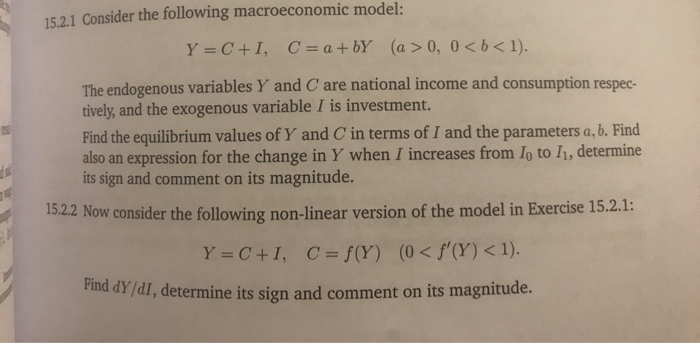15.2.1 Consider the following macroeconomic model: Y = C+1, C = a +by (a > 0, 0<b< 1). The endogenous variables Y and C are national income and consumption respec- tively, and the exogenous variable I is investment. Find the equilibrium values of Y and C in terms of I and the parameters a, b. Find also an expression for the change in Y when I increases from Io to I1, determine its sign and comment on its magnitude. 15.2.2 Now consider the following non-linear version of the model in Exercise 15.2.1: Y = C+1, C = f(Y) (0 < f'(Y) < 1). Find dy/dl, determine its sign and comment on its magnitude.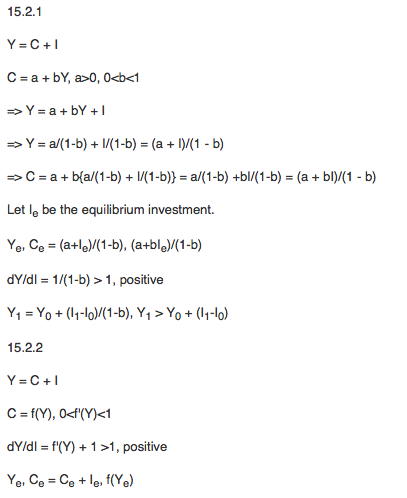#### Earn Coin

Coins can be redeemed for fabulous gifts.

Similar Homework Help Questions
• ### 4. (28 pts) Consider the following macroeconomic model: Y C M = C + Io +...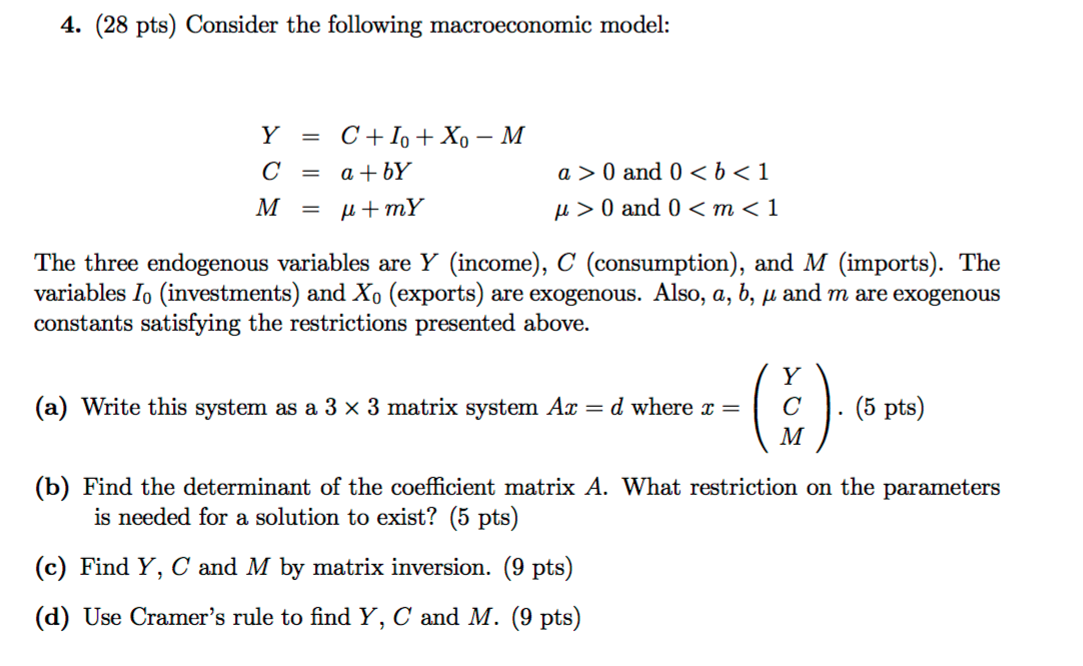4. (28 pts) Consider the following macroeconomic model: Y C M = C + Io + Xo - M = a +bY = u +mY a> 0 and 0 <b<1 u> 0 and 0 <m < 1 The three endogenous variables are Y (income), C (consumption), and M (imports). The variables I. (investments) and X. (exports) are exogenous. Also, a, b, u and m are exogenous constants satisfying the restrictions presented above. (a) Write this system as a 3 x...

• ### . Consider the following macroeconomic model Y C+ C f(Y T) T=qBY where Y is GDP,...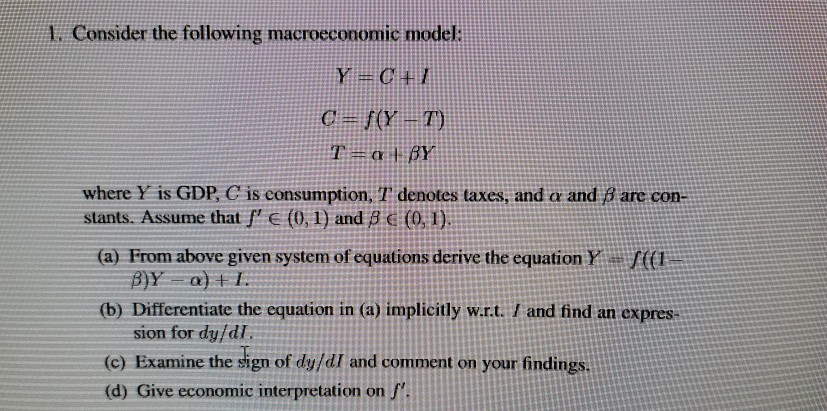. Consider the following macroeconomic model Y C+ C f(Y T) T=qBY where Y is GDP, C is consumption, T' denotes taxes, and a and B are con- stants. Assume that f'€ (0,1) and 8 (0, 1). (a) From above given system of equations derive the equation Y B)Y a)I (b) Differentiate the equation in (a) implicitly w.r.t. I and find an expres- sion for dy/dI (c) Examine the sign of dy/dI and comment on your findings. (d) Give economic...

• ### EC2040-5 Question 2 [40 points] Consider the following macroeconomic model: Y=C+10 + Go c-a+b(Y-T) Where the...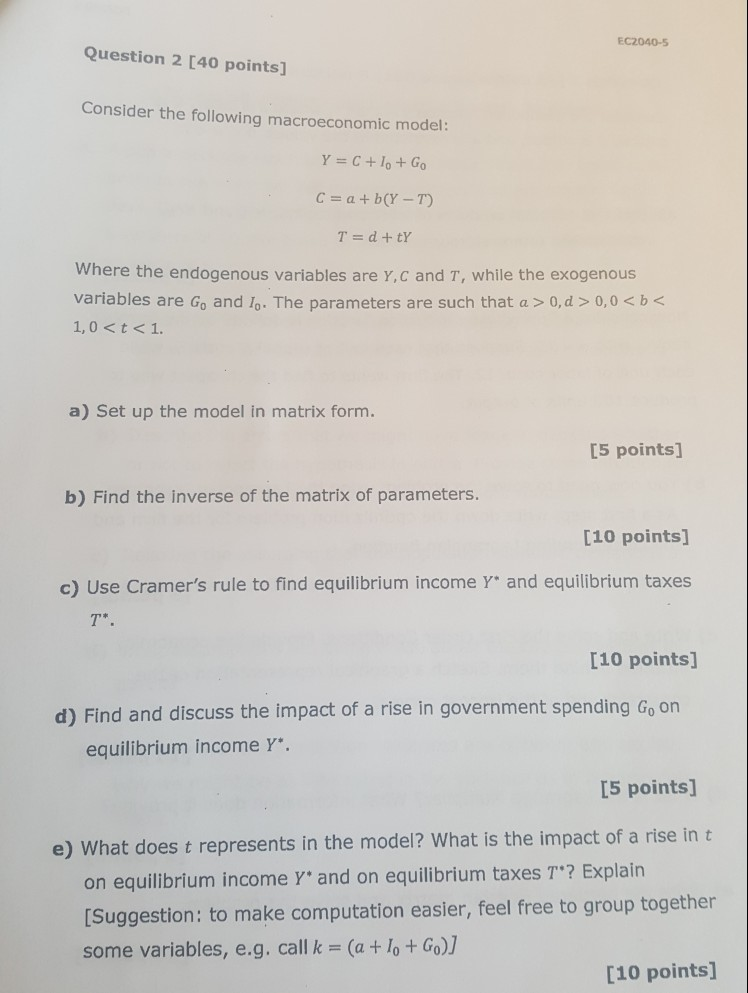EC2040-5 Question 2 [40 points] Consider the following macroeconomic model: Y=C+10 + Go c-a+b(Y-T) Where the endogenous variables are Y,c and T, while the exogenous variables are Go and lo. The parameters are such that a > 0,d 0,0 < b a) Set up the model in matrix form. [5 points] b) Find the inverse of the matrix of parameters [10 points] c) Use Cramer's rule to find equilibrium income Y and equilibrium taxes [10 points] d) Find and discuss...

• ### in the macroeconomics model below matrix In the macroeconomic model below, Y is aggregate output, C...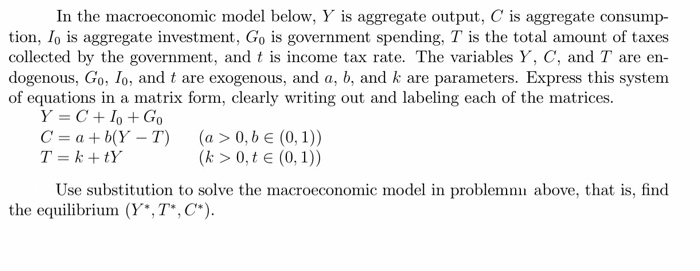in the macroeconomics model below matrix In the macroeconomic model below, Y is aggregate output, C is aggregate consump- tion, Io is aggregate investment, Go is government spending, T is the total amount of taxes collected by the government, and t is income tax rate. The variables Y, C, and T are en dogenous, Go, lo, and t are exogenous, and a, b, and k are parameters. Express this system of equations in a matrix form, clearly wrting out and...

• ### 2. Consider the National Income model given by the following equations. Y-C-I.-G, = 0 C-a-B(Y -...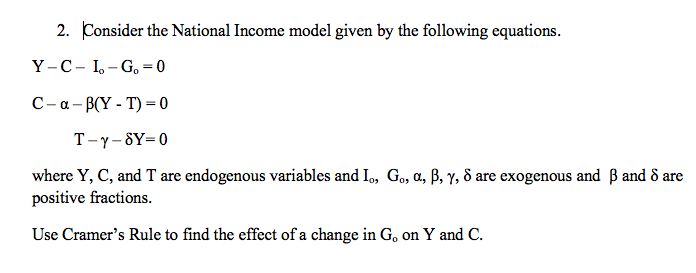2. Consider the National Income model given by the following equations. Y-C-I.-G, = 0 C-a-B(Y - T) = 0 T-y-SY=0 where Y, C, and T are endogenous variables and I., G., a, b, Y, 8 are exogenous and B and 8 are positive fractions. Use Cramer’s Rule to find the effect of a change in G, on Y and C.

• ### 6) In the macroeconomic model below, Y is aggregate output, C is aggregate consump- tion, I....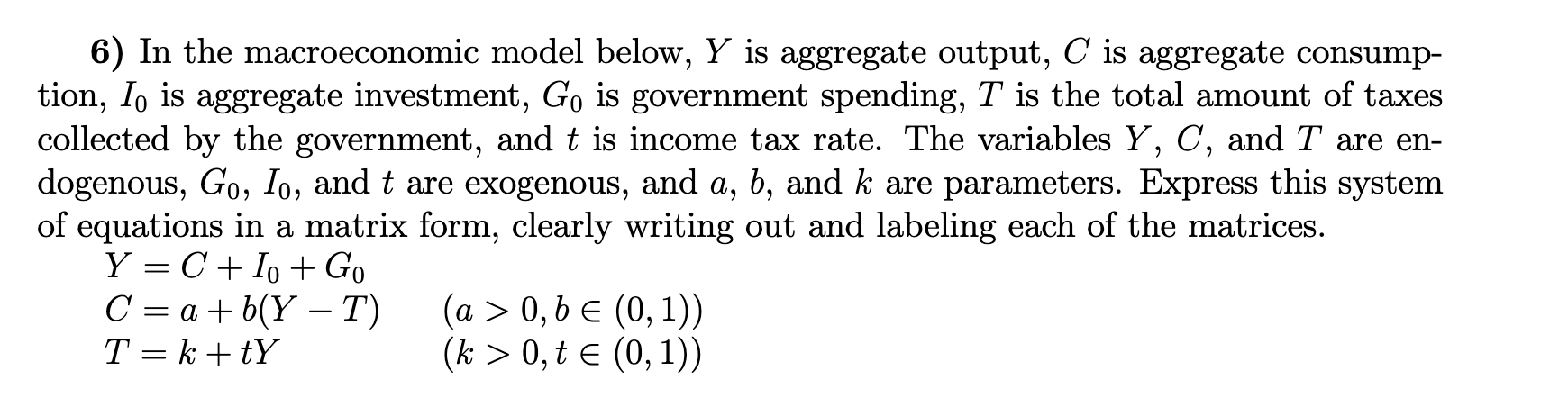6) In the macroeconomic model below, Y is aggregate output, C is aggregate consump- tion, I. is aggregate investment, Go is government spending, T is the total amount of taxes collected by the government, and t is income tax rate. The variables Y, C, and T are en- dogenous, Go, Io, and t are exogenous, and a, b, and k are parameters. Express this system of equations in a matrix form, clearly writing out and labeling each of the matrices....

• ### 6) In the macroeconomic model below, Y is aggregate output, C is aggregate consump- tion, Io...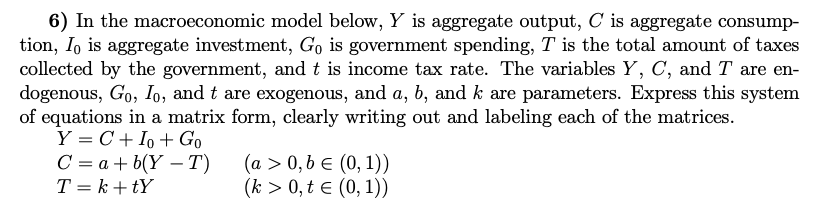6) In the macroeconomic model below, Y is aggregate output, C is aggregate consump- tion, Io is aggregate investment, Go is government spending, T is the total amount of taxes collected by the government, and t is income tax rate. The variables Y, C, and T are en- dogenous, Go, Io, and t are exogenous, and a, b, and k are parameters. Express this system of equations in a matrix form, clearly writing out and labeling each of the matrices....

• ### 6) In the macroeconomic model below, Y is aggregate output, C is aggregate consump- tion, Io...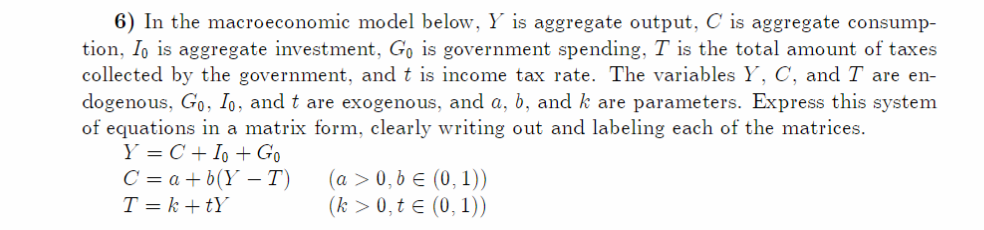6) In the macroeconomic model below, Y is aggregate output, C is aggregate consump- tion, Io is aggregate investment, Go is government spending, T is the total amount of taxes collected by the government, and t is income tax rate. The variables Y, C, and T are en- dogenous, Go, Io, and t are exogenous, and a, b, and k are parameters. Express this system of equations in a matrix form, clearly writing out and labeling each of the matrices...

• ### 4. Points = 18. Consider IS-LM Model: Real Sector: Y=C+I+G C = a +b (1-t) Y...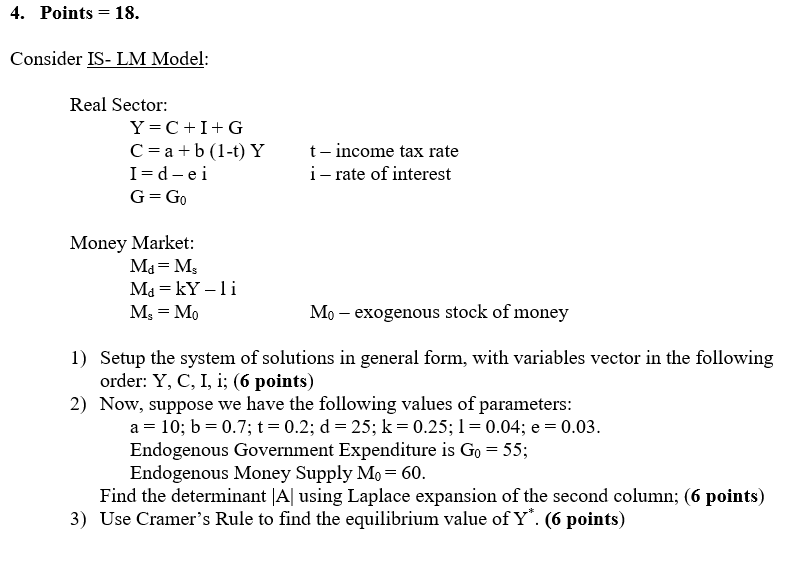4. Points = 18. Consider IS-LM Model: Real Sector: Y=C+I+G C = a +b (1-t) Y I=d-ei G=Go t-income tax rate i-rate of interest Money Market: Ma=M Ma= kY-li Mg = Mo Mo - exogenous stock of money 1) Setup the system of solutions in general form, with variables vector in the following order: Y, C, I, i; (6 points) 2) Now, suppose we have the following values of parameters: a = 10; b = 0.7; t = 0.2; d...

• ### Consider IS- LM Model Real Sector: Y C+IG C ab (1-t) Y I d-e t-income tax...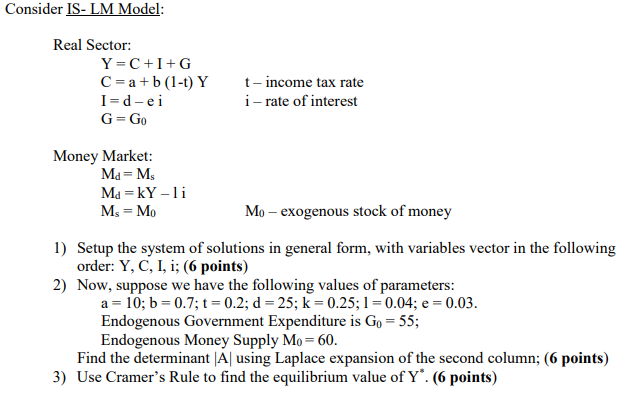Consider IS- LM Model Real Sector: Y C+IG C ab (1-t) Y I d-e t-income tax rate i-rate of interest G Go Money Market: Md Ms Md kY - Ms Mo Mo - exogenous stock of money 1) Setup the system of solutions in general form, with variables vector in the following order: Y, C, I, i; (6 points) 2) Now, suppose we have the following values of parameters: a 10; b 0.7;t= 0.2; d 25; k 0.25;1 0.04; e...